# Multivariate Normal distribution and Cholesky decomposition in Stan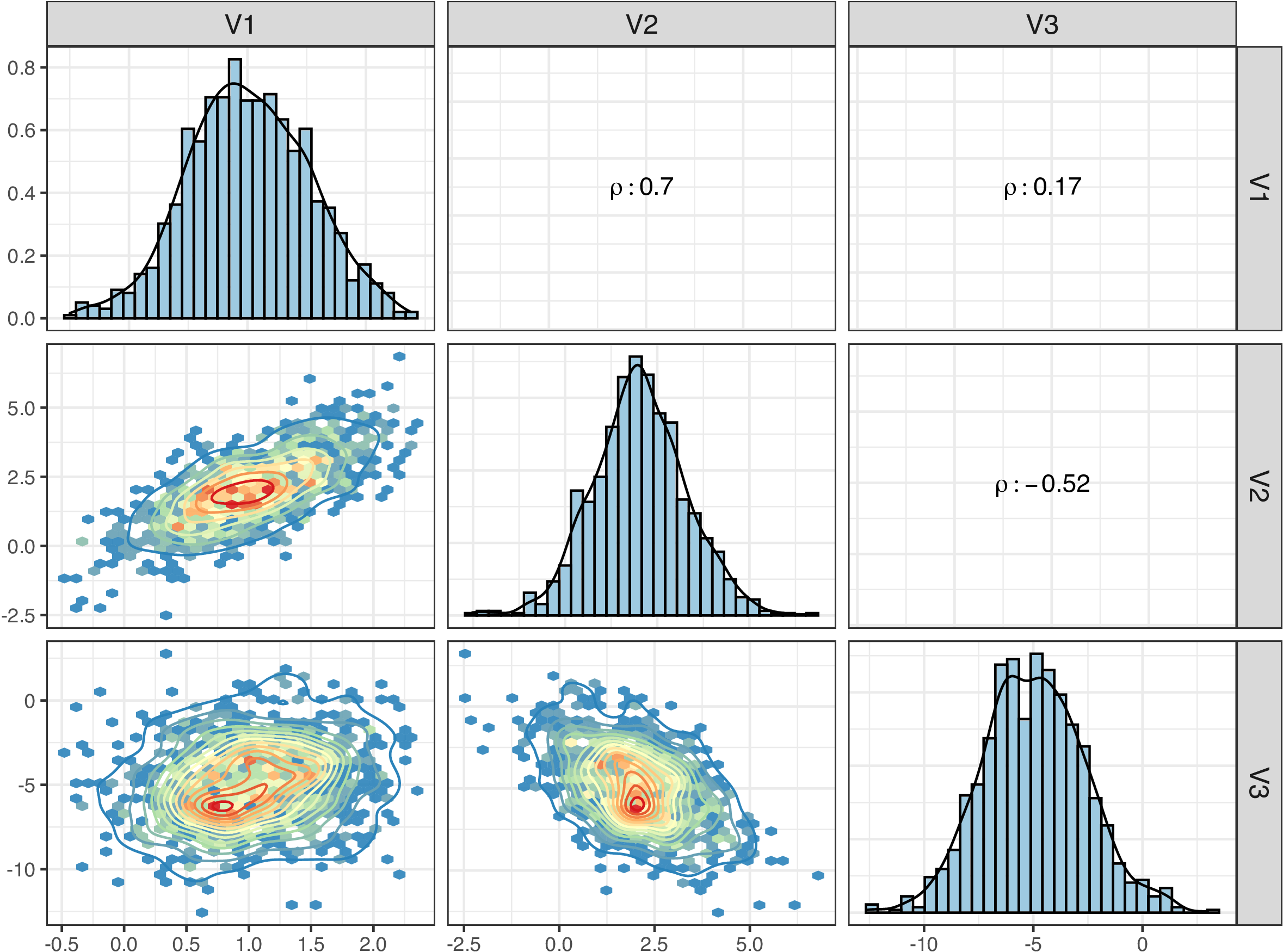Figure 1: Simulated data in a Multivariate Normal distribution

This post provides an example of simulating data in a Multivariate Normal distribution with given parameters, and estimating the parameters based on the simulated data via Cholesky decomposition in `stan`. Multivariate Normal distribution is a commonly used distribution in various regression models and machine learning tasks. It generalizes the Normal distribution into multidimensional space. Its PDF can be expressed as: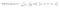PDF of a Multivariate Normal distribution

where mu is a vector of k elements for the location parameters and Sigma is the Variance-Covariance matrix. The |Sigma| calculates the absolute determinant of Sigma in the equation above.

Cholesky decomposition is used to decompose a positive-definite matrix into the product of a lower triangular matrix and its transpose. In our case, we will decompose the correlation matrix (R) into the product of two triangular matrices (L*L’). Note: even though we can directly perform Cholesky decomposition on the Variance-Covariance matrix (Sigma), it is not recommended, since we may fail to estimate the standard deviations (sigma) of each variable.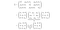Matrix representation of Cholesky decomposition

## 1. Data simulation

The variance-covariance matrix (Sigma) for data simulation and its analytical solution via Cholesky decomposition are provided below. For example, the standard deviation for the first variable sd_1 is 0.5, and the correlation coefficient (rho) between first and second variables is 0.7 (see Figure 1 for more intuitive illustration).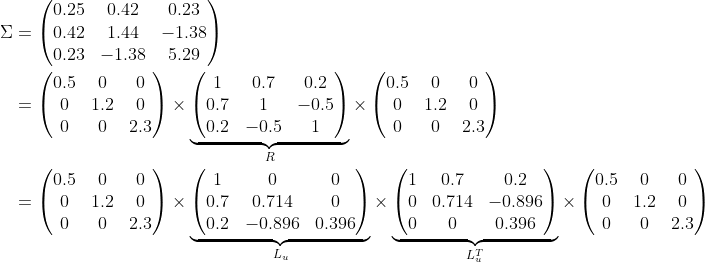Decomposing an example VCV matrix via Cholesky factorization

To generate the data, we are using the mvrnorm function from the package MASS by specifying a vector of means (mu) and variance-covariance matrix (Sigma).

`mu = c(1, 2, -5) # meansR = matrix(c(1, 0.7, 0.2, # correlation matrix (R)             0.7, 1, -0.5,             0.2, -0.5, 1), 3)sigmas = c(0.5, 1.2, 2.3) # sd1=0.5, sd2=1.2, sd3=2.3Sigma = diag(sigmas) %*% R %*% diag(sigmas) # VCV matrixdata = mvrnorm(1000, mu = mu, Sigma = Sigma) # data simulation`

## 2. Parameter estimation in stan

With the pseudodata generated above, we can try to recover the parameters in the multivariate normal distribution. Here we are using a probabilistic programming language, `stan`, to estimate the parameters of the multivariate normal distribution based on the previously simulated data. The `stan`code block is attached below, and you can directly embed it in R.

With the compiled stan model, we can start the sampling process and summarize the estimated parameters.

`input = list(N = 1000, K = 3, y = convertRowsToList(data))results = sampling(stan_MVnorm_model, data=input,                   chains = 4, iter = 2000, cores = 4)`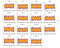Figure 2: Traceplots for the estimated parameters in the Multivariate Normal model

Useful link:

Programming, Data analysis & Deep learning!

Programming, Data analysis & Deep learning!